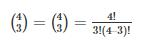Courses

# The number of arrangements of the letters in the word FAILURE, so that vowels are always coming together isa)576b)575c)570d)none of theseCorrect answer is option 'A'. Can you explain this answer? Related Test: Test: Basic Concepts Of Permutations And Combinations- 1

## CA Foundation Question

By Deepak Kumar · Jun 02, 2019 ·CA Foundation
Deepak Kumar answered Aug 30, 2018
There are 4 vovels so we can write statement like this(A,I,U,E)f,L,R now this starement permutation will be 4*3*2*1 but vovels can also rearrange there position so now vovels permutation will be 4*3*2*1 now total arrangements will be 4*3*2*1+4*3*2*1 which is 576

Mayank Sharma answered Nov 06, 2018
here are seven letters in the word ‘FAILURE’ out of which the consonants are- F, L and R. And the vowels are A,E,I and U. There are total 4 odd positions (1st, 3rd, 5th and 7th) and 3 even positions (2nd, 4th and 6th) to fill.

The constraint is that consonants may occupy only an odd position. So, first we’ll fill the odd positions with the consonants. There are total 4 odd positions and 3 consonants. Since there are only 3 consonants, then at a time, 3 consonants can occupy only 3 odd positions and one will be left out. So, we’ll need to select which three odd positions out of the four available odd positions we will fill first. The number of ways of selecting 3 odd positions out of four are:This gives us 4 ways to select 3 odd positions out of the available 4 odd positions. Now that we have selected the odd positions to fill, we now need to arrange the consonants in these positions. The number of ways in which we can arrange 3 consonants is equal to 3.Now only the vowels remain to be arranged. There are 4 vowels and 4 vowels can be arranged in 4!.

Now we multiply these results to arrive at the answer: 4∗3!∗4!=576 ways.

Smilangi Modem answered Jun 06, 2019
576